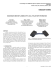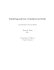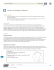# Robotic origami folding - Department of Computer Science

## Transcription

Robotic origami folding - Department of Computer Science
```Robotic origami folding
Matthew T. Mason
Robotics Institute
Carnegie Mellon University
Pittsburgh, PA 15213
[email protected]
Devin J. Balkcom
Department of Computer Science
Dartmouth College
Hanover, NH 03755
[email protected]
Abstract
Origami, the art of paper sculpture, is a fresh challenge
for the field of robotic manipulation, and provides a concrete example for many difficult and general manipulation
problems. This paper describes our initial exploration,
and highlights key problems in manipulation, modeling,
and design of foldable structures. Results include the first
origami-folding robot, a complete fold-sequence planner
for a simple class of origami, and analysis of the kinematics of more complicated folds, including the common
paper shopping bag.
Figure 1: A simplified samurai hat being folded by a robot.
1 Introduction
lation include paper bags, garments, fast-food containers, sheet-metal, car airbags, space-telescope mirrors, and
MEMS. Building products out of thin sheets may reduce
material costs, and allow storage in small volumes.
Origami also reveals limitations of the state-of-the-art
in robotic manipulation. Hardware is one problem. Humans have dozens of degrees of freedom in their hands,
touch-sensitive skin, and capable binocular vision. The
industrial robot that forms the core of our paper-folding
machine has four degrees of freedom, and does not sense
the paper – the robot could be compared to a blind man
with no sense of touch folding origami with one finger.
The primary challenges, however, are algorithmic. We
do not know how to manipulate, model, or design foldable
structures.
The first challenge is minimalist manipulation. Paper
has infinite degrees of freedom, and sensing and control are hard. Occlusion, the thin-ness of the paper, and
the presence of curved surfaces are challenges for vision
Humans are far more skilled than robots at manipulating
flexible, unpredictable materials. The clearest example is
origami, the human art of paper sculpture. Figure 1 shows
the state of the art in robotic origami folding – a simplified
samurai hat being folded by a robot in our lab. Figure 2
shows Icarus, folded by master Hojyo Takashi out of a
single piece of paper, without cutting or gluing.
This paper examines origami from the perspective of
robotic manipulation. There are many compelling reasons to explore and better understand folding manipulation, and origami provides a useful starting point.
A better understanding of techniques for designing and
folding flexible structures would be of great practical use.
In the past, automated manufacturing with rigid bodies
was the driving application for the study of robotic manipulation; tasks include grasping, fixturing, pushing, sorting, and feeding. Applications of deformable manipu1
closed chain.
Traditional sampling-based path planners struggle with
environments containing narrow corridors. The configuration space of a closed chain may be a union of several
manifolds, containing infinitely thin corridors. The probability that a random-sampling planner will find a path between two points on different manifolds is zero, if every
path must contain points on a connecting region of lower
dimension.
In this paper, we present a few configuration-space parameterizations that allow local planning, and discuss geometric techniques for analyzing the global topological
structure of the configuration space.
The third challenge is design. Not all patterns of
creases fold equally well. For example, we show that
the common paper shopping bag cannot be folded without flexing or bending the paper in regions where there
are no creases. How complicated a model is necessary to
describe the folding of a shopping bag, and can creases
be added so that the bag folds predictably? Ultimately,
we want to design software that can automatically create
crease patterns that allow one shape to be folded into another smoothly, while maximizing rigidity at the initial
and final configurations.
Figure 2: An example of the state-of-the-art in human folding:
Icarus, by Hojyo Takashi; dry-folded out of a single piece of
paper. Photograph used by permission.
or laser-range-finding. Tactile sensors are even worse –
touching the paper is likely to deform it.
Humans have some tricks for manipulating paper in
spite of the sensing difficulty. Figure 3 shows an example. The goal is to fold a precise diagonal crease. The
folder grasps two corners and brings them into precise
alignment. The fingers of one hand then flatten the bulge
in the paper. Since the paper does not stretch, a crease
forms at the extreme region of the paper, along the diagonal. The fingers extend and sharpen the crease. The process requires minimal sensing, with only a few degrees
of control. We would like to build robots that use similar
techniques, but our first attempt is much more crude: the
robot places creases using a vice-like clamp that flattens
the paper near the crease.
The second challenge is modeling. Even if we model
folding paper as a collecting of rigid facets connected by
hinges at the creases, the configuration space of a foldable structure may be complicated. The simplest model of
creased paper is a collection of rigid bodies with hinges.
If creases meet at a vertex, the mechanism is a kinematic
1.1
There are many levels of origami complexity. The simplest traditional origami designs require only sequential straight-line folds. At the next level of complexity,
birds, frogs, and the waterbomb require multiple creases
that meet at a vertex to be manipulated simultaneously,
while modern three-dimensional insects and flowers require multi-vertex networks of creases to be manipulated simultaneously. State-of-the-art origami sculpture
requires even more complicated techniques. Masks require bending facets and folding curved creases, animal
sculptures are often folded using wet paper, and modular
origami requires assembly of several pre-creased sections.
Figure 4 shows our current state of progress. The simplest skills can be implemented on a robot; we have built
a robot and automatic planning software to fold simple
origami, including paper airplanes, an origami cup, and a
simplified samurai hat.
We understand more advanced skills less well. For example, the (unsimplified) samurai hat requires that two
2
Figure 3: Creating a valley fold using landmarking.
Path existence
Manipulation skill
Modeling
A few theorems
A robot
Local planning
C-space topology Complete planning
Simple folds
Mountain
Valley
Reflection folds
Degree-4 single-vertex folds
Reverse folds
Prayer folds
Petal folds
Squash folds
Degree-n single-vertex
Crease networks
Curved creases
Sinks
Flat
Pureland
Classification
Crumpling, wet-folding
Modular origami
Origami sculpture
skills
Figure 4: A map of the origami task domain.
coplanar sections of paper be separated so that a flap can
be folded. We have built a planner to explore the possible fold-sequences for the samurai hat, but the robot cannot reliably separate facets of paper. The paper crane is
yet more complicated; the paper must be precreased and
unfolded to create a pattern where multiple creases meet
at a vertex. These creases must be manipulated simultaneously, as shown in figure 11. The mechanism is a
kinematic closed chain, and motion planning for closed
chains is a well-known open problem in robotic manipulation. We can describe local parameterizations of the
configuration space that allow local planning, and have
techniques for analyzing the global structure of the con-
figuration space, but do not have a complete planner.
We know very little about the most sophisticated
origami. We can build mathematical models of origami
with curved creases, networks of creases, or curved sections of the paper, but have only studied the simplest of
examples.
The structure of the paper follows the map described
in figure 4 vertically, from simple folds through crease
networks.
3
1.2 Related work
Box folding and sheet metal bending are the two robotics
applications closest to origami folding; see Lu and
Akella [Lu and Akella, 1999, Lu and Akella, 2000],
Liu and Dai [Liu and Dai, 2003], and Gupta et
al.
[Gupta et al., 1998].
In
preliminary
work [Balkcom, 2004, Balkcom and Mason, 2004],
we focus on the simplest possible model of origami:
rigid bodies connected by hinges at the creases.
Miyazaki et al.’s [Miyazaki et al., 1992] software simulates simple origami manipulation under this model,
and a rigid-body model for cartons with origamilike folds has also been studied by Dai, Rees Jones,
and Liu [Dai and Jones, 2002b, Dai and Jones, 2005,
Dai and Jones, 2002a, Dai and Jones, 1999].
When creases intersect, even the simplest model poses
challenges, since the mechanism is a closed chain. Motion of closed chain mechanisms can be simulated efficiently (see Ascher and Lin [Ascher and Lin, 1999]),
and the configuration-space topology of spherical closed
chains of the type found in origami has been analyzed by
Kapovich and Millson [Kapovich and Millson, 1995]; our
approach is based on work on planar closed chains by Milgram and Trinkle [Milgram and Trinkle, ming].
In fact, the kinematics of origami mechanisms may provide inspiration for new mechanism designs, as suggested by Rodrigues-Leal and
Dai [Rodrigues-Leal and Dai, 2007].
One of the interesting properties of paper is
that it bends but does not stretch; such surfaces
are said to be developable; Hilbert and CohnVossen [Hilbert and Cohn-vossen, 1952] is a good
reference.
Several authors have used developable
surfaces to approximate the state of paper and
cloth, including Redont [Redont, 1989], Sun and
Fiume [Sun and Fiume, 1996],
Leopoldseder and
Pottmann [Leopoldseder and Pottmann, 1998], Pottmann
and Wallner [Pottmann and Wallner, 1999], Weiss
and Furtner [Weiss and Furtner, 1988], and Aumann [Aumann, 1991].
Huffman [Huffman, 1976]
considers creases as limiting cases of developables,
particularly networks of creases and curved creases.
Sometimes creases occur because there are constraints
applied that are inconsistent with the paper remaining a smooth developable surface; see Kergosien et
Figure 5: A sequence of two simple folds.
al [Kergosien et al., 1994]. Dynamic simulation of cloth
and paper is an active research area in the graphics community. Baraff and Witkin’s [Baraff and Witkin, 1998]
work is seminal; Choi and Ko [Choi and Ko, 2002]
and Bridson et al [Bridson et al., 2002] present recent
approaches.
There is a rich field of work on origami design in the mathematics community; Demaine et
al. [Demaine and Demaine, 2001] provides a survey.
Robert Lang’s papers and TreeMaker software [Lang, 2001] and Hull [Hull, 1994] are usually
credited with being the first in-depth work.
1.3
Piecewise-rigid origami
Although the mechanics of folding require that paper
bend, it is useful to consider a very simple model of
origami composed of rigid polygonal facets connected by
revolute joints at the creases. Define the origami pattern
to be the placement of the creases on unfolded origami.
Creases meet at interior vertices of the pattern; if n creases
meet, we say that a vertex is of degree n. The angles between creases around a vertex in the pattern are called
sector angles.
Each crease connects two facets. We associate with
each pattern a facet graph, whose nodes are facets and
edges are creases. Any tree that spans the facet graph is a
facet tree. Facet trees are easy to construct; any complete
search method such as breadth-first or depth-first search
is suitable.
A facet tree implies a parent-child relationship between
two facets connected by a crease. We will choose the
convention that all facets are described by a counterclockwise set of points in the pattern; we will associate
a unit vector with each crease such that the vector’s direc4
tion agrees with the order of vertices in the child facet. We
then describe the crease angle as the angle between a parent facet and its child; the sign is chosen to be consistent
with the right-hand rule applied to the crease vector.
Given a pattern and any facet tree, the crease angles
associated with all uncut creases determine the configuration of the origami mechanism – the pose of each facet
and the angle of each cut crease can be determined by
traversing the facet tree applying rotations to descendent
facets.
Since origami can be folded essentially flat, it is convenient to allow crease angles in the range [−π, π]. The
order in which facets are folded becomes important when
crease angles reach extreme values and facets become
coplanar. We will call a group of coplanar facets a compound facet. With each compound facet we associate a
normal vector and a partial ordering of facets that describes the order in which the compound facet may be assembled or disassembled: the facet stacking. The height
of a facet is its height in the stacking, and the height of a
crease is the height of its child facet.
What can be folded using a sequence of simple folds?
Figure 7 shows two examples: a simple paper cup, and a
simple paper airplane.
The design of the machine is based on the observation
that it is not necessary to flip the paper over at any step, if
the sequence of folds is planned carefully:
Fact 1 Any origami piece that can be folded by a sequence of flips and simple folds can be folded by a single
initial flip and a sequence of valley simple folds.
Proof: A mountain simple fold is equivalent to a flip,
valley simple fold, flip sequence; write m = f vf . Second, f f is the identity. Third, either the facets on the
left or on the right of the crease line can be chosen as the
base. Using similar notation, vl f = vr , and vr f = vl .
These substitution rules imply that any fold sequence can
be rewritten to include only a single initial flip and a sequence of valley simple folds. First, remove all mountain
folds from the sequence. Then remove all f f . Then each
flip except the first is preceded by a valley fold. Remove
the flips by changing the direction of each of these valley
folds.
The most obvious limitation of the machine is that simple folds cannot be used to separate two co-planar flaps of
paper. In some cases, careful pre-planning can help with
this problem, too. A human being would probably fold
the body of the airplane shown in figure 7 first, and separate and fold the wings down as the last step. However,
by folding the wings first, as shown in steps 4 and 5, this
separation step can be avoided. Section 3 will discuss an
automatic fold-sequence planner for simple origami.
2 Simple folds: an origami-folding
machine
The most basic origami fold takes all paper on one side of
a crease line and folds it to the other side. Figure 5 shows
a sequence of two simple folds, described using the rigid
origami model, and figure 3 shows a human executing a
simple fold. Figure 6(a) shows a machine designed to
allow a 4 DOF SCARA robot arm to make simple folds.
The folding procedure is outlined in figure 6(b). The
arm grasps the paper using a vacuum pad, and positions
the paper over the folding mechanism. A blade presses
the paper into a slot in the folding mechanism (step 2);
friction holds the paper in the slot as the blade is removed.
The slot clamps shut, forming the crease (step 3). Steps
4 and 5 show a method for removing the paper from the
slot and placing it flat on the table; this is required since
the arm only provides one rotational degree of freedom at
the wrist. First the blade sweeps across the paper, forcing
it to lie flat. The clamp is released while the blade holds
the paper against the table; the springiness of the paper
allows it to swing free of the slot.
3
Reflection folds and fold-sequence
planning
The foldings of the hat and paper airplane were planned
automatically by a complete sequence planner for simple
origami. The input to the planner is the origami pattern
and the desired final stacking of the facets in the folded
state. The output is a sequence of folds to make, and a
set of configurations where the robot arm must place the
paper for each fold.
The algorithm is a simple breadth-first search – the flat
pattern is the root node of the search tree, and children
5
(a) The design.
(b) Making a simple fold with the machine; side view.
Figure 6: A machine that can fold simple origami.
Figure 9: Two designs that can be reflection-folded but not
simply-folded.
The algorithm is unfortunately exponential in the number of creases in the pattern. Some efficiency can be
gained by storing intermediate configurations and pruning
branches of the tree that reach previously-explored configurations; this is described in more detail in section 3.2.
Figure 7: Simple foldings of two traditional origami designs.
of each node are generated by enumerating all possible
simple folds.
Given a flat origami state, what simple folds are possible? First, find the minimal set of lines that contains all
creases. Discard any crease lines that cross a facet. Each
remaining crease line divides the facets into two compound facets; we arbitrarily assign one to be the ‘base’
and one the ‘flap’. During folding, the base will not move.
The flap can folded either up (a valley simple fold) or
down (a mountain simple fold).
To execute the fold, all creases colinear with the crease
line are folded simultaneously. During folding, the
heights of facets in the flap are reversed, and then either
stacked above or below the base, forming a single new
compound facet.
3.1
Reflection folds
Figure 9 shows two examples of origami that cannot be
simply folded. We define a reflection fold: any fold for
which all the active creases are colinear such that the continuous rigid-body rotation of the moving facets does not
cause self-intersection of the origami or tear any creases.
We will call the moving facets the flap, and the fixed facets
the base. All facets lie in a plane both before and after the
fold.
The simple folds from an origami state are easy to enumerate. Finding the possible reflection folds is somewhat
more complicated. The following observation is useful to
limit the number of possibilities that must be considered.
6
Figure 8: Robotic folding of a paper hat. Subfigures a) through d) show the first crease, e) through i) the second, j) through m) the
third, n) through q) the fourth, r) through u) the fifth, and v) and x) show the final product – a folded hat.
7
Fact 2 The set of active creases in any fold cuts the facet
graph, separating each pair of relatively moving compound facets.
Proof: Choose two compound facets that move relative to one another; call one the base, and one the flap.
Any crease that connects the base and flap and is colinear
with the crease line must be active; the crease angle will
be the angle between the base and flap, up to sign. Any
crease that connects the base and flap but is not colinear
with the crease line will be torn by any rotation of the flap
around the crease line.
We can determine the set of all reflection folds from
an origami state, using the following algorithm. First,
enumerate all crease lines. Sort the creases by height
in the stacking. Consider all sequential combinations of
creases that contain either the minimum- or maximumheight crease. Test each of these crease sets to determine
if it cuts the facet graph into at least two pieces. All pieces
strictly to the left of the current crease line that do not
include the root node of the facet tree (which is always
fixed) are candidate flaps, as are all pieces strictly to the
right. (For simplicity, we do not consider combinations of
pieces of the graph as candidate flaps, since combinations
can be folded by a sequence of reflection folds.) Test each
candidate flap to see if and in which direction(s) folding is
possible without self-intersection of the origami; this can
be accomplished by polygon intersections in the plane of
the compound facet.
The state of the origami after a reflection fold is easy
to determine: reflect the flap across the crease line, flip
flap stacking, and stack the flap either above or below the
base, depending on the direction of the fold.
Figure 10: Automatically-planned folding of the samurai hat.
With the exception of the reflection fold in step 7, all folds are
‘simple’.
planner for reflection-foldable origami; the nodes of the
graph are flat origami states. Figure 10 shows an automatically planned folding of the samurai hat comprised
of eight simple folds and one reflection fold.
The input to the planner is the pattern and the desired
stacking of the facets. The algorithm is as follows. Use
the goal stacking and the pattern to determine signs on
the crease angles. Insert the pattern into the search queue
as the initial state. While the search queue has elements,
pop, test for goal state, and if goal, backchain to find the
plan. Otherwise, determine the reflection folds from the
state and generate successor states. Cull any states that
have crease angles that do not agree with those of the goal
state. Also cull any states that have been previously visited. Insert remaining states into the visited list and into
the search queue.
The visited list is implemented as a hash table that
hashes on the integer heights of facets in the stacking.
Before testing against the visited list, the compound facet
of the state is collapsed to determine a minimal stacking.
The algorithm to find the minimal stacking is essentially a
bubble sort – each facet is allowed to bubble downwards
in the stacking as long as it does not intersect with any
facets in the level underneath it.
3.2 Reflection-fold sequence planning
The planner implementation is about 5000 lines of C++
Origami that can be folded using reflection folds is flat af- code, and was run on a 500 mhz Pentium III. The table
ter each fold. Since the motion of the flap occurs out of the below shows results for four traditional origami designs.
plane of the base, collision detection is only necessary at
the beginnings and ends of folds, and only requires simple
creases nodes CPU time (sec) folds
Origami
polygon intersection tests. Futhermore, the origami state
Cup
9
30
.1
5
after each fold is just the stacking of the facets, together
Airplane
9
24
.1
5
with the set of creases that have been folded, and is thus
14
75
.5
5
Hat
discrete. (Note that crease angles can be determined from Samurai hat
20
4250
110
9
the stacking, as long as we know which creases have been
For the samurai hat, more than 99% of the CPU time
folded.) We have implemented a complete graph search
8
m
Figure 11: Frames from an animation of the initial ‘prayer fold’
of a crane.
n
Figure 12: Huffman’s notation for a degree-four vertex.
was spent in polygon intersections to determine minimal up’ and one ‘elbow-down’. Huffman [Huffman, 1976]
derives a relationship between opposite crease angles m
stackings and to find reflection folds.
and n for degree-four origami,
4 Kinematics of rigid origami
1 − cos n =
Many origami designs cannot be reflection folded; figure 11 shows an example. Inside and outside reverse
folds, squash folds, and petal folds all manipulate four
creases simultaneously.
Analysis of foldability requires that we consider both
the kinematics of degree-n folds and the possibility of
self-intersection. It is well-known that piecewise-rigid
origami with a single vertex has the kinematics of a spherical linkage. The kinematics of degree-four spherical linkages are understood, and we draw on some of these results
to further show that self-intersection can only occur when
the origami is folded completely flat.
For higher-degree vertices, and origami patterns containing several vertices connected by a network of creases,
the problem is more challenging. We present a parameterization of the configuration space for these more complicated mechanisms, but the parameterization describes
only the local motion of the mechanism, and not the
global structure of the configuration space. In section 5
we present a graphical method for determining this global
structure, and analytical results based on Milgram and
Trinkle’s work on the topology of the configuration spaces
sin A sin B
(1 − cos m),
sin C sin D
(1)
where A, D, C, and B are sector angles as shown in figure 12.
The sequence planner for reflection folding described
above relies on a key observation – self-intersection can
occur only when the origami is flat. We can use Huffman’s formula to show that a similar result holds for
origami where four creases that intersect at a vertex are
manipulated simultaneously.
To show this, we need a simple lemma:
Lemma 1 Continuous motions of degree-four origami
mechanism cannot cause self-intersection without at least
one joint angle reaching either zero or π.
internal angle between them reaching 0. Pick a link; call
it the base. (See figure 13.) Call the endpoints of the
first occur when a or b is coplanar with the base. If a is
coplanar with the base, then the joint angle between the
base and the adjacent link containing a must be one of 0
or π; if b, then the joint angle between the base and the
If we assume that the origami design is such that it can
be folded flat, a stronger result holds:
4.1 Self-intersection around degree-four
vertices
If one of the crease angles is known, then there are up Theorem 1 Rigid flat-foldable degree-four origami can
to two possible configurations of the paper, one ‘elbow- only self-intersect when flat.
9
Figure 13: A ‘cut’ degree-four origami mechanism.
Figure 14: Solving for three dependent crease angles.
Proof: Assume that there is a collision. From
lemma 1, at least one crease angle is 0 or π. Label the
mechanism so that crease angle is m, and label the sector
angles A, B, C, and D as shown in figure 12.
For flat-foldable origami, Kawasaki’s theorem constrains A + C = π and B + D = π; thus for flat-foldable
origami, there exists an integer i s.t. n = m + iπ. Since
m is 0 or π at a collision, n is an integer multiple of π.
Since at least creases m and n are folded flat, facets A
and B are coplanar, as are C and D. Let (AB) be the compound facet containing A and B, and (CD) be the compound facet containing C and D. If these compound facets
are coplanar, we are done. If they are not, the structure is
that of a simple pair of hinged planes that is collision-free
unless flat. (In fact, this a a ‘simple’ fold.)
possible using the inverse kinematics approach described
in [Han and Amato, 2000].)
Figure 14 shows the procedure; ϕ1 , ϕ2 , and ϕ3 are the
crease angles to be solved for. First cut the crease corresponding to ϕ3 , and flatten the paper. For any valid configuration of the paper, the two cut edges must ‘line up’
in such a way that they could be re-glued together. Let pl
and pr be points along these edges a unit distance from
the vertex.
Anchor the facet clockwise from the ϕ3 crease, and
choose a coordinate system with origin at the vertex and
with the x-axis along the ϕ1 crease. The point pr lies at
a fixed position within the z = 0 plane in this coordinate
system.
If pl were permitted to move, then its location would be
given by a sequence of rotations about each of the creases.
Let Rx and Rz be matrices describing rotation about the
4.2 Single-vertex origami
x- and z axes respectively. Let R1 , R2 , and R3 be maMore advanced origami skills require the simultaneous trices corresponding to rotations about the independent
manipulation of more than four creases. In the remainder crease angles, as shown in figure 14.
of the section, we present the relationship between crease
The closure constraint can now be written as
angles for vertices of arbitrary degree; our result is also
applicable to the case where there is missing or excess
R1 Rx (ϕ1 )R2 Rz (α)Rx (ϕ2 )Rz (−α)R3 pl = pr , (2)
sector angle around the vertex.
The mobility of a vertex of degree n is n − 3. Our goal is to solve for ϕ1 and ϕ2 , given R1 , R2 , and
We will therefore choose n − 3 arbitrary independent R3 , which may be easily computed from the indepencrease angles as input, and solve for the remaining dent crease angles and the geometry of the paper. Rewrite
crease angles. (In the special case where the depen- equation 2:
Rx (ϕ1 )ZRx (ϕ2 )a = b,
(3)
dent crease angles are sequential, a simpler solution is
10
where Z, a, and b may be computed:
Z = R2 Rz (α)
(4)
a = Rz (−α)R3 pl
(5)
b=
R1T pr .
(6)
Multiplying out equation 3 gives three equations, the first
of which is
k3 = k1 cos ϕ2 + k2 sin ϕ2 ,
(7)
with k1 , k2 , and k3 computed to be
k1 = z12 a2 + z13 a3
(8)
k2 = z13 a2 − z12 a3
k3 = b1 − z11 a1 .
(9)
(10)
Figure 15: A multi-vertex pattern with a mobility of five.
If k1 = k2 = 0, then equation 7 implies that ϕ2 can 5 The topology of origami configutake on any value. Otherwise, equation 7 has the soluration space
tion(s)
!
The parameterizations described in section 4.2 allow simk3
ϕ2 = atan(k2 , k1 ) ± acos p 2
.
(11)
ulation and local planning for origami and other spherical
k1 + k22
There may be zero, one, two, or infinitely many solutions
for ϕ2 . For each value of ϕ2 , the remaining two rows
of equation 3 can be used to solve for ϕ1 , which either
has a unique value or is unconstrained. ϕ3 is uniquely
determined by the angle between the normals to the facets
at either end of the cut chain.
1. The configuration of a rigid origami mechanism is
completely determined by the dihedral angles, but
not all choices of dihedral angles satisfy the constraints imposed by the geometry of the paper and
the crease pattern.
2. The parameterizations are not global: the mapping
from certain input joint angles to output joint angles
may be one-to-many.
4.3 Multi-vertex origami
For a single vertex of degree n, we can view n − 3 of
the creases as ‘inputs’, and 3 of the creases as ‘outputs’.
3. Finding a trajectory from start to goal that satisfies
Given the dihedral angles at the input creases, equation 11
the constraints can be difficult. The space of configand the results of the previous section can be used to comurations may have multiple components, or sections
pute the dihedral angles at the output creases.
of the configuration space may be joined only at speSome folds require that multiple connected vertices be
cific regions along their boundaries. Parameterizamanipulated simultaneously. Figure 15 shows a network
tions give no information about the connectedness of
of four vertices.
configuration space.
An output from one vertex can be viewed as the input to the adjacent vertex. Therefore, any labeling of the
This section describes the connectedness and topology
creases as either output or input that satisfies the property
that each vertex has three outputs can therefore be used of configuration spaces of n-bar spherical closed chains.
to construct a local parameterization of the configuration The analysis uses techniques described in Milgram and
Trinkle [Milgram and Trinkle, ming].
space, and simulate local motion of the crease network.
11
Figure 16: Four flat configurations of a square piece of paper
with two diagonal creases, and the topological structure of the
associated configuration space.
5.1 Four- and five-bar mechanisms
Figure 17 shows an example, for the case where the first
two sector angles counterclockwise from the horizontal
are equal. We first cut the paper along one of the creases,
as shown. If the crease angles were known for creases 1
and 2, then the configuration of the mechanism would be
completely determined. However, there is an additional
constraint – that the crease angles of the uncut creases be
such that the edges of the cut crease ‘line up’. We will
therefore analyze the behavior of a point on the cut crease
(points A and B in the figure), and see how it restricts
motion of the other creases.
We label the creases as shown in figure 17, cut crease
3, and rigidly attach the facet between creases 1 and 4 to
the ground. Consider the motion of the point A as the
paper is allowed to fold along creases 1 and 2. Point A
is a fixed distance from the central vertex, and can move
on the surface of a sphere. Its motion is also bounded
on the left by a plane normal to crease 1, and containing
point A. There are two configurations of crease angles 1
and 2 that allow point A to reach most locations on the
sphere: crease 2 may be convex, or concave. There are
some locations that can only be reached in one way: those
that fall on the plane normal to crease 1 and containing
point A. There is also one point that can be reached in an
infinite number of ways, at the intersection of crease 1 and
the sphere.
Now consider point B, that rotates around crease 4. The
reachable locations form a circle that lies in a plane perpendicular to crease 4.
If the cut is removed, point A and point B must touch;
we will call this point AB. AB must move on the intersection of the sphere cut by a plane that A moves on, and the
circle that B moves on. The locations that AB can reach
therefore form an arc of a circle.
We can describe the space of possible configurations of
the paper by the ways in which point AB can reach each
point on the arc. There are two configurations that reach
each point on the interior of the arc (crease 2 may be either
concave or convex). There is only one way in which each
of the endpoints of the arc can be reached – crease 2 is flat
at each endpoint.
Each point on the arc corresponds to a slice of the space
of configurations of the paper, described by crease angles
1 and 2. Starting at one endpoint of the arc, the slice is a
single configuration. Moving continuously along the arc,
each new slice corresponds to two configurations. At the
final slice (at the other endpoint of the arc), there is only
one configuration. The topology of this shape, and thus
of the configuration space, is a circle – a 1-dimensional
manifold with one component.
In general, the set of reachable locations of point A is a
sphere bounded by two planes perpendicular to crease 1.
The intersection of this surface with the circle reachable
by point B can be a circle, an arc of a circle, or two arcs
of a circle. Depending on the shape of this workspace,
and the ways in which point AB can reach each point on
the workspace, the configuration space may have one of
several different structures, as shown in figure 18.
12
• Null intersection. One side of the circle may be completely contained in the workspace. The pre-image
of an arc completely contained within the workspace
is two arcs.
• Transverse intersection. One side of the circle may
be cut by the bounding plane at two points. The preimage of an arc touching the bounding plane is an
arc.
• Tangent intersection. The circle just touches a
bounding circle of non-zero radius. The pre-image
of an arc tangent to the bounding circle is a pair of
arcs touching at a single interior point.
• Radius-zero intersection. The circle touches the
bounding plane at one of the poles of the sphere on
the x axis. The pre-image of this point is a circle of
the x axis; the pre-image of an arc through this point
is two arcs connected by a circle.
• We ignore the case where the circle is completely contained within the boundary of the open
workspace.
Figure 17: A degree-four vertex, cut along crease 3.
Five-bar mechanisms may be analyzed by fixing one
of the dihedral angles, analyzing the resulting four-bar
mechanism, and considering how the topology of the
four-bar configuration space changes as the (initially)
fixed dihedral angle is varied.
5.2
null-null
transverse-transverse
As can be seen from the analysis of four-bar mechanisms
in the previous section, the structure of the configuration
null-tangent
transverse-tangent
space origami can be very complicated, even if we ignore
self-intersections. In order to design a motion planner,
or to write an algorithm that determines if paths between
null-transverse
transverse-null
two configurations exist, we would like to know whether
the configuration space is a manifold, where it branches
into separate sections, and if there are lower-dimensional
tangent-null
regions that connect different sections of the space.
The idea behind the graphical analysis of the topology
tangent-tangent
of configuration spaces is to cut the mechanism and anaradzero-tangent
lyze an open chain with n − 2 revolute joints to determine
the ways that the open chain can reach each point in the
tangent-transverse
workspace. We then consider the curve of points, that the
endpoint of the remaining chain, with one revolute joint,
can reach. The pre-image of the forward kinematics map
for the (n − 2)-joint arm at each point on that curve correradzero-radzero
sponds to a slice of the configuration space for the mechanism. The topology of these slices only changes at critical
(or singular) configurations of the arm.
In this section, we more formally analyze the configuraFigure 18: Thirteen of the sixteen possible ways a circle can intion
space in terms of the singularities and the workspace
tersect the workspace of an open three-bar spherical chain. For
each class, the ellipses on the left show the workspace; the cir- of an open spherical chain, using tools from Morse thecles on the right show the configuration space (the pre-image of ory. In this analysis, we ignore joint limits and selfthe workspace). There are seven distinct topological classes of intersections.
configuration space.
We have not developed a practical planning algorithm
for high-degree-of-freedom origami closed chains, but the
13
theorem and corollaries in this section yield some insight into the structure of the configuration space, W .
The theorems and proofs essentially track Milgram and
Trinkle’s[Milgram and Trinkle, ming] results for planar
and spatial closed chains with ball joints, which they have
used to design a complete motion planner for those systems.
A possibly more complete, analysis of the configuration space of origami mechanisms is presented by
Kapovich and Millson [Kapovich and Millson, 1995]; our
method has the advantage of a relatively simple geometric interpretation, and a graphical method for determining
whether the configuration space is a manifold.
Theorem 2 describes the singularities, or critical points,
of the forward kinematics map for the (n − 2)-joint chain,
in terms of the crease angles. Corollary 1 describes the
image of these critical points on the sphere on which the
endpoint of the cut chain can move: a set of circles all
perpendicular to the first axis. (The circle containing the
point A in figure 17 is an example of such a circle, but in
longer chains, there may also be several circles interior to
the workspace of A.)
Corollary 2 then considers the relationship between the
workspace of the (n−2)-joint chain, and the configuration
space of the entire origami mechanism, W . Specifically,
we let γ be the curve traced by the single-link mechanism
(the circular arc traced by B, in figure 17; the configuration space W is the pre-image of the intersection of γ and
the workspace of the (n−2)-joint chain under the forward
kinematics map. Specifically, we show that the configuration space is a manifold iff γ intersects each critical circle
transversally.
The following definitions are taken from Milnor [Milnor, 1997]. We say that a map between two manifolds is smooth if all of the partial derivatives exist and are
continuous. Consider a smooth map f : M 7→ N , from a
manifold of dimension m to a manifold of dimension n.
Let C be the set of all x ∈ M such that
dfx : T Mx 7→ T Nf (x)
has rank less than n (is not onto). Then C will be called
the set of critical points, f (C) the set of critical values,
and the complement N − f (C) the set of regular values
of f .
Consider the forwards kinematics map f : M 7→ N
from the torus M = S 1 × S 1 × . . .× S 1 of dihedral angles
to the workspace of an endpoint on the last facet. The map
can be written as a product of rotation matrices applied to
the initial location of the endpoint, and is smooth.
The workspace N may be constructed iteratively. Spin
the endpoint around the m − 1 axis, creating a circle with
radius dependent on sector angle βm−1 . Call this circle
Nm−1 . Spin the circle around the m − 2 axis; call the result Nm−2 . Since all axes intersect at the origin, Nm−2 is
a section of a sphere, bounded by two half-planes perpendicular to the m − 2 axis. Spin each resulting workspace
around the preceding axis. The workspace N is equal to
N1 , and is the intersection of the unit sphere S 2 with two
halfspaces with normals pointing along the first axis. N
is therefore either a two-manifold or a two-manifold with
boundary.
Theorem 2 Consider an open spherical chain with all
sector angles B0 . . . Bn−1 less than π. The critical points
of the forwards kinematics map which sends a configuration of the chain to its endpoint are the configurations
for which the first dihedral angle ranges over [0, 2π), and
each of the remaining dihedral angles is one of {0, π}.
Proof: The configurations of the system can be described by a list of vectors corresponding to the current
location of the endpoint Xn and each axis Xi in the
workspace,
q = (X1 , X2 , . . . , Xn ),
(12)
with the constraints
||Xi || = 1
∠Xi Xi+1 = βi .
(13)
(14)
The linear map dfθ between the tangent spaces of the
torus and the workspace can be described by the Jacobian
of f . Use the cross-product method to write the Jacobian:
Jf = [ X1 × Xn
| . . . | Xn−1 × Xn ]
(15)
If X1 . . . Xn lie in a plane, then the Jacobian has rank
less than two, and the configuration is a critical point.
Proof of the converse: X1 and X2 are linearly independent, so at least one column of the Jacobian (either
X1 × Xn or X2 × Xn ) is non-null. All axes and the endpoint must lie in a plane perpendicular to this column.
Since X1 . . . Xn lie in a plane iff all of the dihedral angles except the first are one of {0, π}, this completes the
proof.
14
| X2 × Xn
Corollary 1 The critical values of f are the circles
formed by rotating the points (cos ρk , sin ρk ) around the
X1 axis, where k ranges over 0 . . . 2n−1 , and
ρk =
n−1
X
(−1)ei−1 (k) βi ,
(16)
i=1
with ei (k) denoting the ith bit of k.
Lemma 1 from Milnor [Milnor, 1997] states that if
f : M 7→ N is a smooth map between manifolds of dimension m ≥ n, and if y ∈ N is a regular value, then
the set f −1 (y) ⊂ M is a smooth manifold of dimension
m − n.
Corollary 2 Let γ be a curve on the unit sphere that intersects critical circles only at discrete points, and that
does not contain any critical circles of radius 0, and let
W = f −1 (γ). W is a differentiable manifold if and only
if γ intersects each critical circle transversally.
Figure 19: A shopping bag with the traditional crease pattern.
Proof: Assume the curve γ is described by a pair of
constraints of the form
p(x, y, z) = 0
2
2
2
x + y + z = 1,
(17)
where p has the property that its gradient ▽p is normal to
the unit sphere.
The algebraic variety W is a subset of the torus of dihedral angles, and can described by the composition of the
constraints described by equation 17 with the forwards
kinematic map f . Since the forwards kinematic map already constrains the endpoint to lie on the unit sphere,
along any path θ(t) contained in the variety W ,
d
p(f (θ(t))) = ▽pT f˙ = ▽pT Jf θ̇ = 0.
dt
Edge vertex
Corner vertex
Figure 20: The three types of vertex found in a shopping bag.
cross products will be linearly independent, and the rank
of JW is therefore one. At a critical point of f where γ is
transverse to the critical circle, ▽p makes a non-zero dot
product with X1 × Xn , and the rank of JW is one. At a
critical point of f where γ is tangent to the critical circle,
every dot product is zero, and JW is degenerate.
(19)
6
So the Jacobian of the variety W is
JC = ▽pT Jf
= ▽pT (X1 × Xn )
Side vertex
(18)
(20)
. . . ▽p (Xn−1 × Xn ) .
(21)
T
An example of 3D (non)foldability: the paper shopping
bag
The
Bellows
Theorem,
proven
in
1997
The Jacobian has only one row, and describes the nor- [Connelly et al., 1997] states that “any continuous
mal to W . At any regular point of f , at least two of the flex that preserves the edge-lengths of a closed triangu15
cannot be folded by bending facets.
C
C
C
C
S
S
E
Fact 3 A piecewise rigid shopping bag with the traditional crease pattern cannot be continuously folded between the open and closed states.
E
Figure 21: The vertex graph for a shopping bag. The nodes represent ‘edge’, ‘side’, and ‘corner’ vertices, and the edges represent creases that connect vertices.
lated surface of any genus in three-space must flex in
such a way that the volume it bounds stays constant.”
This implies that no polyhedron with a fixed, finite
number of creases is sufficient to model the deflation of
a closed airbag, or the inflation of a teabag or origami waterbomb. But where are the boundaries? What origami
structures can or cannot be satisfactorily modeled with
fixed crease patterns?
In this section, we consider what is probably the most
commonplace origami-like structure: the paper shopping
bag. Perhaps surprisingly, it turns out that a shopping bag
with rigid facets, and creases in the usual places, cannot
be folded flat. Specifically, the bag has a configuration
space that is just isolated points corresponding to the flat
and fully open states. This might be considered a design
feature; since the facets resist bending and crinkling, the
bag tends to stay in its current configuration, either open
or closed.
Figure 19 shows the traditional crease pattern for a
shopping bag. The height of the bag is h, the width is
w, and the depth is d. We assume that h > d/2; this ensures that the diagonal creases on the right and left sides
of the bag meet.
We can distinguish three types of vertex; see figure 20. The vertices in the middle of each of the
right and left sides of the bag have sector angles of
(90◦ , 135◦ , 90◦ , 45◦ ). There is a vertex along each of the
two of the upright edges of the bag, with sector angles
(90◦ , 90◦ , 90◦ , 90◦ ). There are vertices at the corners of
the bag with sector angles (90◦ , 90◦ , 45◦ , 45◦ ).
Some pairs of vertices share a crease; figure 21 shows
how vertices of each type are connected to one another.
We can use equation 1 to show that the shopping bag
Proof: Consider an ‘edge’ vertex. There are four π/2
sector angles, so equation 1 implies hat the two vertical
creases that meet at this vertex have crease angles that are
equal in magnitude. Assume that the magnitude of these
crease angles is 0 or π. In this case, it is easy to show by
traversing the vertex graph and applying 1 that the bag is
flat, and we are done.
If the magnitude is not 0 or π, then the two horizontal
creases from this vertex must be one of {0, π}. Choose
a crease that is 0 or π, and connected to another vertex.
Walk the crease network; each of the left and right sides
is flat (open or folded), and each of the corners is either
fully open or collapsed.
The following observations can be made:
1. A shopping bag with creases in the ‘usual’ places is
rigid.
2. Two shopping bags taped together at their tops cannot be flattened with a finite number of fixed creases.
(Thanks to Robert Lang for this example of the bellows theorem.)
3. A shopping bag cannot be turned inside-out with a finite number of fixed creases. (According to Erik Demaine, is has been proven by Connelly that a convex
vertex cannot be turned inside out using a finite number of creases, but this work may not yet have been
published.) Robert Lang points out that this work
implies that the ‘closed sink’ origami move that inverts a convex vertex cannot be modeled with a finite
number of creases.
A natural question is whether a shopping bag can be
folded by adding a finite number of creases. With Erik
and Martin Demaine, we have shown that it can, and a
future paper will discuss this and other problems of 3D
foldability.
16
7 Conclusion
[Balkcom, 2004] Balkcom, D. J. (2004). Robotic origami
folding. Ph.D. Thesis, published as Carnegie Mellon
The paper has presented the first origami-folding robot,
University RI TR 04-43.
and an initial exploration into issues regarding folding
[Balkcom and Mason, 2004] Balkcom, D. J. and Mason,
manipulation.
M. T. (2004). Introducing robotic origami folding. In
There are several promising directions for future work,
IEEE International Conference on Robotics and Auincluding far more capable machines for folding, and
tomation, pages 3245–3250.
mathematical tools for analyzing more general models of
foldability.
We are particularly interested in ‘low-level’ manipulation skills (like landmarking) that humans use to precisely
and reliably place creases in flexible paper. We also intend to explore further aspects of complex closed-chain
manipulation. The configuration spaces of closed chains
can be complicated, and understanding connectedness of
these c-spaces is still an open problem.
Another interesting problem is understanding multivertex patterns like that shown in figure 15. Although we
have presented a simple parameterization of the configuration space for multi-vertex patterns, topological analysis to determine the connectedness of such spaces seems
to be a challenging problem.
Acknowledgments
[Baraff and Witkin, 1998] Baraff, D. and Witkin, A.
(1998). Large steps in cloth simulation. In SIGGRAPH, pages 43–54.
[Bridson et al., 2002] Bridson, R., Fedkiw, R., and Anderson, J. (2002). Robust treatment of collisions, contact, and friction for cloth animation. In SIGGRAPH.
[Choi and Ko, 2002] Choi, K.-J. and Ko, H.-S. (2002).
Stable but responsive cloth. In SIGGRAPH.
[Connelly et al., 1997] Connelly, R., Sabitov, I., and
Walz, A. (1997). The bellows conjecture. Contributions to Algebra and Geometry, 38(1):1–10.
[Dai and Jones, 1999] Dai, J. and Jones, J. R. (1999).
Mobility in metamorphic mechanisms of foldable/erectable kinds. Transactions of ASME: Journal
of Mechanical Design, 121(3):375–382.
Thanks to Erik and Martin Demaine, Robert Lang, Zhong
You, Jeffrey Trinkle, Douglas James, and James Kuffner [Dai and Jones, 2002a] Dai, J. and Jones, J. R. (2002a).
Carton manipulation analysis using configuration
for their many discussions and contributions to the present
transformation. Journal of Mechanical Engineering
work. The graphical method described in section 5.1 is
Science, 216(5):543–555.
based on discussions with James Milgram.
The work of Devin Balkcom was supported by a De[Dai and Jones, 2002b] Dai, J. and Jones, J. R. (2002b).
partment of Energy Computational Science graduate felKinematics and mobility analysis of carton folds in
lowship. Funding was further provided by NSF grants IIS
packing manipulation based on the mechanism equiv0082339 and IIS 0222875.
alent. Journal of Mechanical Engineering Science,
216(10):959–970.
References
[Dai and Jones, 2005] Dai, J. and Jones, J. R. (2005).
Matrix representation of topological configuration
[Ascher and Lin, 1999] Ascher, U. and Lin, P. (1999).
transformation of metamorphic mechanisms. TransSequential regularization methods for simulating meactions of the ASME: Journal of Mechanical Design,
chanical systems with many closed loops. SIAM Jour127(4):837–840.
nal on Scientific Computing, 21(4):1244–1262.
[Demaine and Demaine, 2001] Demaine, E. D. and De[Aumann, 1991] Aumann, G. (1991). Interpolation with
maine, M. L. (2001). Recent results in computational
developable Bézier patches. Computer Aided Geometorigami. In Proceedings of the 3rd International Meetric Design, 8:409–420.
ing of Origami Science, Math, and Education.
17
[Gupta et al., 1998] Gupta, S., Bourne, D., Kim, K. K., [Lu and Akella, 2000] Lu, L. and Akella, S. (2000).
and Krishnan, S. S. (1998). Automated process planFolding cartons with fixtures: A motion planning apning for robotic sheet metal bending operations. Jourproach. IEEE Transactions on Robotics and Automanal of Manufacturing Systems.
tion, 16(4):346–356.
[Han and Amato, 2000] Han, L. and Amato, N. M. [Milgram and Trinkle, ming] Milgram, R. J. and Trin(2000). A kinematics-based probabalistic roadmap
kle, J. (forthcoming). The geometry of configurations
method for closed chain systems. In Workshop on the
spaces for closed chains in two and three dimensions.
Algorithmic Foundations of Robotics, pages 233–246.
Homology, Homotopy, and Applications.
[Hilbert and Cohn-vossen, 1952] Hilbert, D. and Cohn- [Milnor, 1997] Milnor, J. (1997). Topology from the Difvossen, S. (1952). Geometry and the Imagination.
ferentiable Viewpoint. Princeton Landmarks in MatheChelsea.
matics. Princeton University Press.
[Huffman, 1976] Huffman, D. (1976). Curvature and
[Miyazaki et al., 1992] Miyazaki, S., Yasuda, T., Yokoi,
creases: A primer on paper. IEEE Transactions on
S., and Toriwaki, J. (1992). An interactive simulation
Computers, C-25(10):1010–1019.
system of origami based on virtual space manipulation.
[Hull, 1994] Hull, T. C. (1994). On the mathematics of
In Proceedings of the IEEE International Workshop on
flat origamis. In Congressus Numerantium, volume
Robot and Human Communication, pages 210–215.
100, pages 215–224.
[Pottmann and Wallner, 1999] Pottmann, H. and Wall[Kapovich and Millson, 1995] Kapovich, M. and Millner, J. (1999). Approximation algorithms for develson, J. (1995). On the moduli space of polygons in
opable surfaces. Computer Aided Geometric Design,
the Euclidean plane. Journal of Differential Geometry,
16:539–556.
42(1):133–164.
[Redont, 1989] Redont, P. (1989). Representation and
[Kergosien et al., 1994] Kergosien, Y. L., Gotoda, H.,
deformation of developable surfaces. Computer Aided
and Kunii, T. L. (1994). Bending and creasing virtual
Design, 21(1):13–20.
paper. In IEEE Computer Graphics and Applications,
[Rodrigues-Leal and Dai, 2007] Rodrigues-Leal, E. and
pages 40–48.
Dai, J. (2007). From origami to a new class of cen[Lang, 2001] Lang, R. (2001). Trees and circles: an eftralized 3-DOF parallel mechanisms. In Proceedings
ficient algorithm for origami design. In Proceedings
of the 31st ASME Mechanisms and Robotics Conferof the 3rd International Meeting of Origami Science,
ence.
Math, and Education.
[Leopoldseder and Pottmann, 1998] Leopoldseder,
S. [Sun and Fiume, 1996] Sun, M. and Fiume, E. (1996). A
technique for constructing developable surfaces. In
and Pottmann, H. (1998). Approximation of develGraphics Interface, pages 176–185.
opable surfaces with cone spline surfaces. Computer
Aided Design, 30:571–582.
[Liu and Dai, 2003] Liu, J. and Dai, J. (2003). An approach to carton-folding trajectory planning using dual
robotic fingers. Robotics and Autonomous Systems,
42:47–63.
[Weiss and Furtner, 1988] Weiss, G. and Furtner, P.
(1988). Computer-aided treatment of developable surfaces. Computers and Graphics, 12(1):39–51.
[Lu and Akella, 1999] Lu, L. and Akella, S. (1999).
Folding cartons with fixtures: A motion planning approach. In IEEE International Conference on Robotics
and Automation.
18
```

### Contents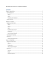### Using Origami to Engage, Promote Geometryаа Understanding, and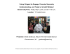### Origami - Leigh Yawkey Woodson Art Museum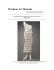### ORIGAMI – Folded Plate Structures, Architecture - Infoscience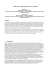### In This Issue - the Hillel Torah North Suburban Day School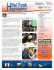### Continuously Flattening Polyhedra Using Straight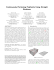### RANCANGAN PELAJARAN TAHUNAN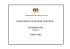### Brochure - Cerwin Vega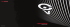### Rhino-Rack Folding Kayak Carrier S512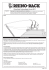### FOR IMMEDIATE RELEASE Press Contact: Marilyn White 973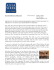### Snakeboard Motion Planning With Local Trajectory Information